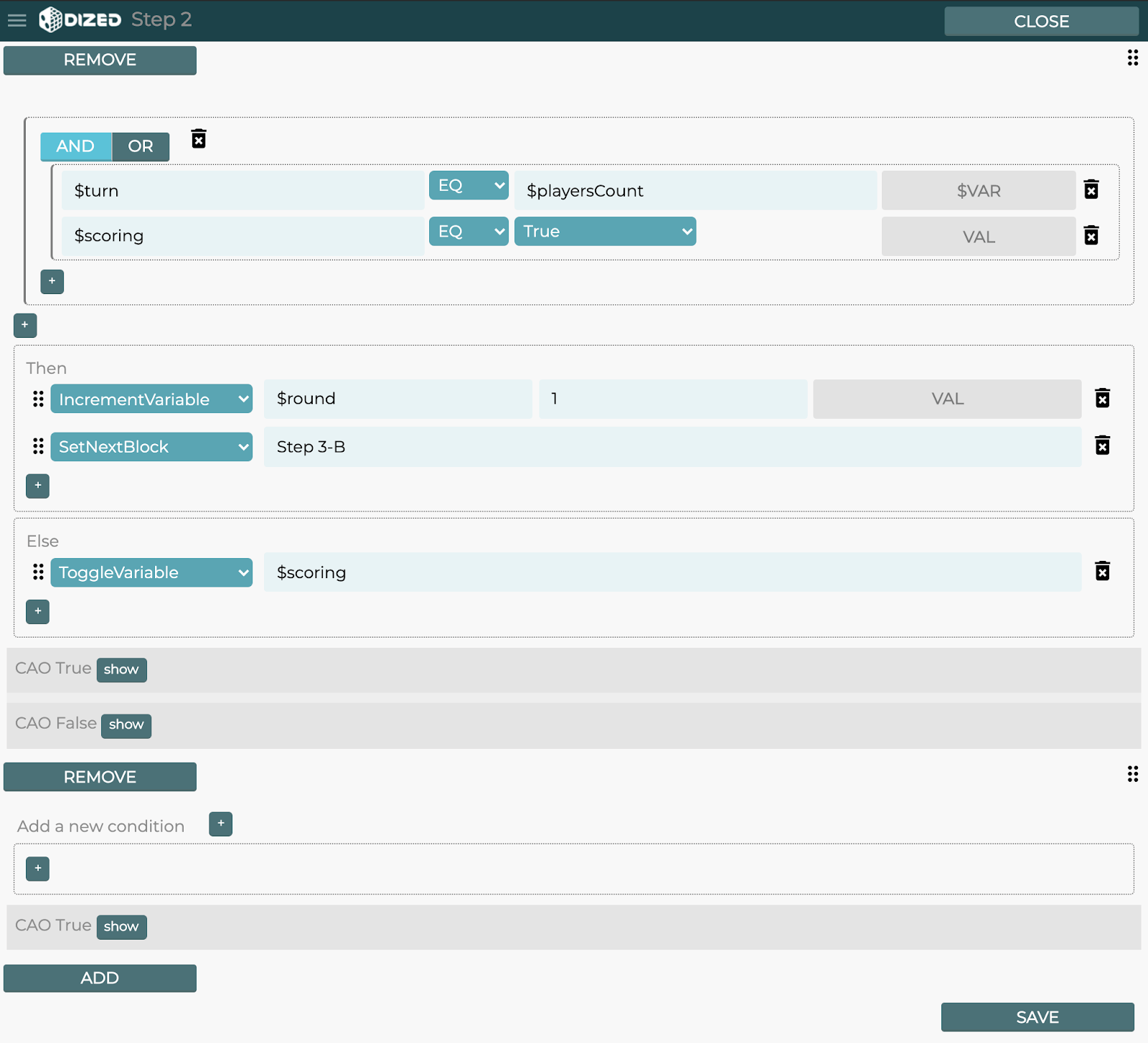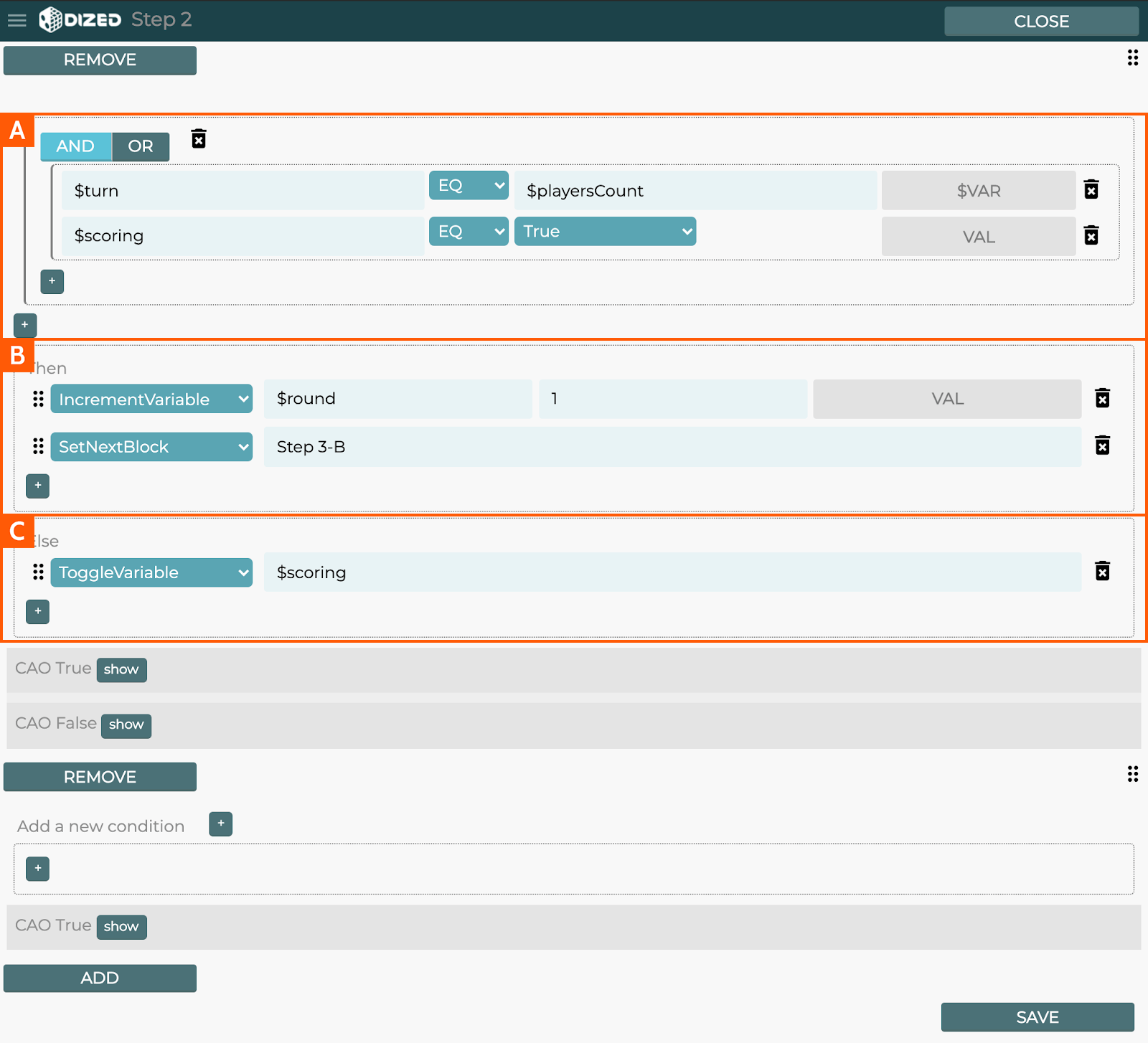# Logic Editor – Overview

The Logic Editor is accessed by double clicking a logic step. Logic steps allow the usage of Actions and Conditions and are not seen by the user.

Each Action is one task, like change the value stored in a variable or go to a step. Conditions simply allow actions to be run depending if the conditions are True (or False).

Conditions and Actions are run from top to bottom. With certain actions it’s possible to cancel the rest of the logic from being run.## How to Read the Logic Editor

See the example below that checks to see if the current round is finished or if the player should be prompted to score their turn:

1. #### IF these are True

IF the value stored in variable \$turn EQUALS the value stored in variable \$playersCount AND IF the value stored in variable \$scoring EQUALS the value True

2. #### THEN do these

THEN increase the value of \$round by 1 AND set the next step to be “Step 3-B”

3. #### ELSE, do that

ELSE flip the value of \$scoring between True and False